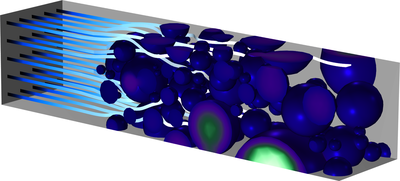# Application Gallery

## Homogenizing a Heterogeneous Electrode Model

Application ID: 92231

In this example, a heterogeneous NMC (Nickel-Manganese-Cobalt) electrode structure is generated from tomography data using a Model Method.

Time-dependent discharge and electrochemical impedance spectroscopy (EIS) simulations are then made on the full 3D geometry.

A solid mechanics simulation is also made to study the effect of electrode expansion/contraction on the particle and binder stresses.

By simulating the effective (volume averaged) flux through the porous structure when solving for the Laplace equation, an effective transport parameter factor is derived, which is then used to homogenize the 3D model into a 1D (+1D extra dimension) model.

Comparisons between the two models are made with regards to the discharge voltage curves and the EIS Nyquist plots.This model example illustrates applications of this type that would nominally be built using the following products: